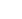# Factorial logistic regression

A factorial logistic regression is used when there two or more categorical independent variables but a dichotomous dependent variable

1. Multiple logistic regression/ Multinomial regression

It is used to predict a nominal dependent variable given one or more independent variables.

When running a multiple regression, one needs to separate variables into covariates and factors. SPSS will automatically classify continuous independent variables as covariates and nominal independent variables as factors. Therefore, when dealing with ordinal independent variables you must choose if you will consider them as covariates or factors.

1. Click Analyze- Regression –Multinomial Logistics
1. Transfer the dependent variable into the Dependent box, the nominal variable into the Factor(s) box and the Covariate(s) box
• The default action SPSS does is to select the last category as the reference category.
1. Click on the Statistic button.
1. Click the Cell probabilities, Classification table, and Goodness-of-fit checkboxes.
2. Click the continue button to return to the Multinomial Logistic Regression dialogue box
3. Click the Ok button to generate the output.

The output window generates a Goodness- of- Fit table, the first row presents the Pearson chi-square statistic. If the P value is less than 0.05 (p<.05) indicate the model does not fit the data well.

Another way to check the measure your model is to consider the Model Fitting Information table. Check the Final row p-value “sig” value or the pseudo R2 measures.

Automated page speed optimizations for fast site performance